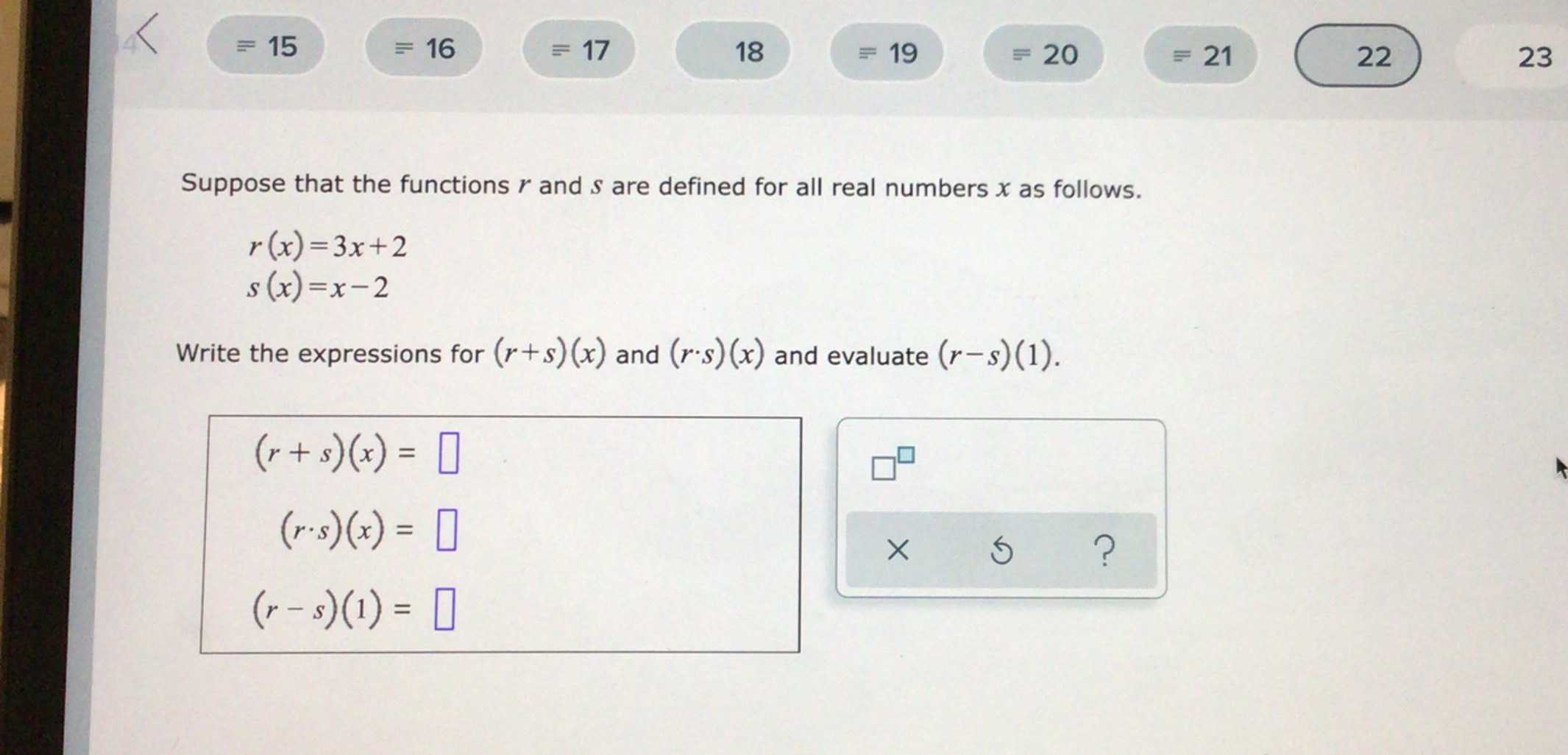### Still have math questions?

Algebra
QuestionSuppose that the functions $$r$$ and $$s$$ are defined for all real numbers $$x$$ as follows.

$$r ( x ) = 3 x + 2$$

$$s ( x ) = x - 2$$

Write the expressions for $$( r + s ) ( x )$$ and $$( r \cdot s ) ( x )$$ and evaluate $$( r - s ) ( 1 )$$

$$( r + s ) ( x ) = \square$$

$$( r \cdot s ) ( x ) = \square$$

$$( r - s ) ( 1 ) = \square$$

(r*s)(x)=$$3x^{2}- 4x- 4$$